trustle > EngliSea > Corona Safe Schooling > mathematics > Quick Math Index > 10 Arithmatic

The study of numbers, especially the properties of the traditional operations on them—addition, subtraction, multiplication and division.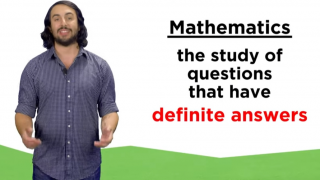01 Introduction to Mathematics
○ Many students wonder why it's even necessary to learn in the first place. ○ How social media knows who you're going to tag in a photo before you do.
481702 Addition and Subtraction
despise, inventory, anthropology, conceptualization, vendor, commutative, associative
4818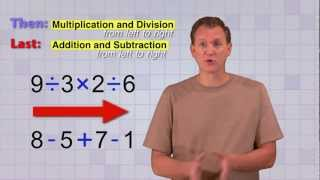04 Order Of Operations
Math Antics - Order Of Operations
5137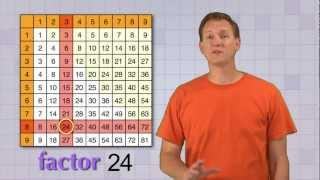05 Factoring
Math Antics - Factoring
5138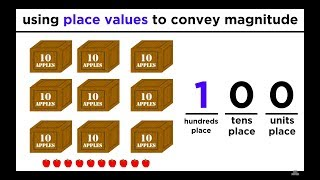05 Large Whole Numbers: Place Values and Estimating
Large Whole Numbers: Place Values and Estimating
4821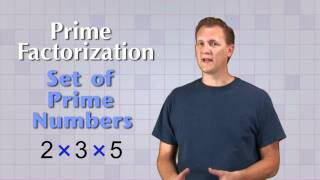06 Prime Factorization
Math Antics - Prime Factorization
5139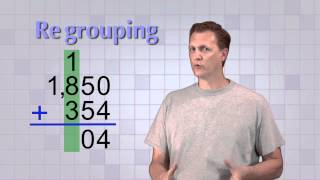07 Multi-Digit Addition
5140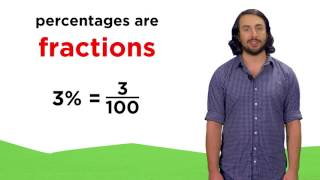07 Working With Percentages
Working With Percentages
4823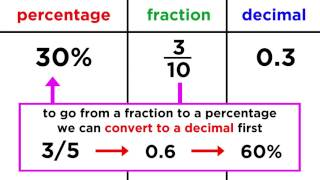08 Converting Between Fractions, Decimals, and Percentages
Converting Between Fractions, Decimals, and Percentages
4824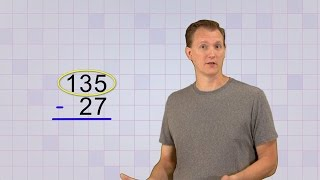08 Multi-Digit Subtraction
Math Antics - Multi-Digit Subtraction
5141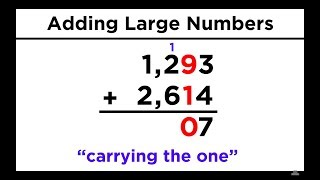09 Addition and Subtraction of Large Numbers
Addition and Subtraction of Large Numbers
4825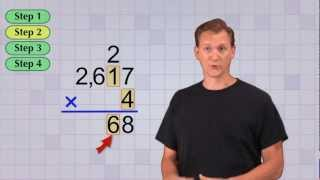09 Multi-Digit Multiplication Pt 1
Math Antics - Multi-Digit Multiplication Pt 1
5142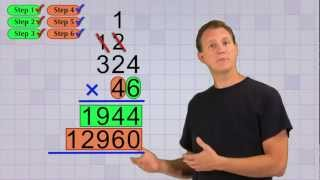10 Multi-Digit Multiplication Pt 2
Math Antics - Multi-Digit Multiplication Pt 2
5143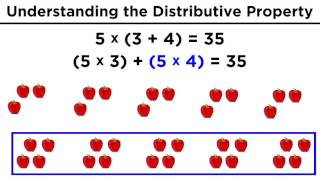10 The Distributive Property
The Distributive Property for Arithmetic
4826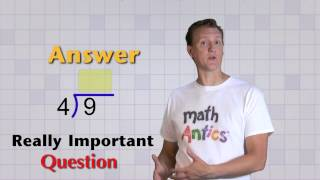11 Basic Division
Math Antics - Basic Division
5144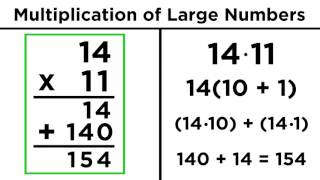11 Multiplication of Large Numbers
Multiplication of Large Numbers
4827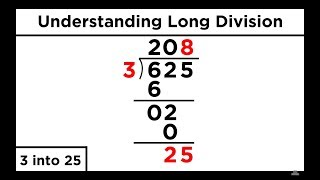12 Division of Large Numbers
Division of Large Numbers: Long Division
4828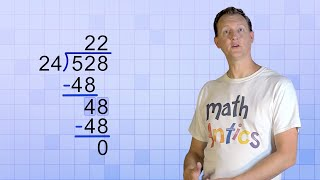13 Long Division with 2-Digit Divisors
Math Antics - Long Division with 2-Digit Divisors
5146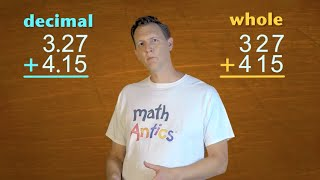14 Decimal Arithmetic
Math Antics - Decimal Arithmetic
5150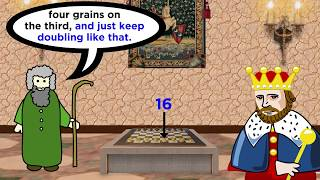14 Understanding Exponents and Their Operations
Understanding Exponents and Their Operations
4830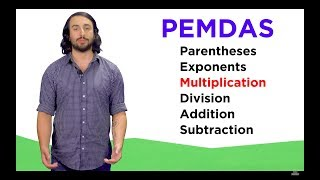15 Order of Arithmetic Operations: PEMDAS
Order of Arithmetic Operations: PEMDAS
4831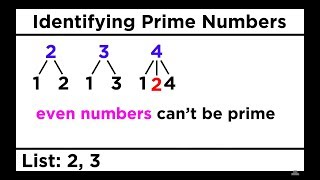16 Divisibility, Prime Numbers, and Prime Factorization
The fundamental theorem of arithmetic: there is only one unique set of prime factors for any number.
4832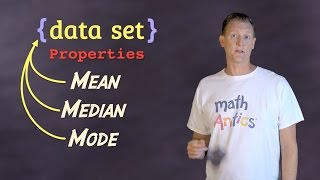16 Mean, Median and Mode
Math Antics - Mean, Median and Mode
5152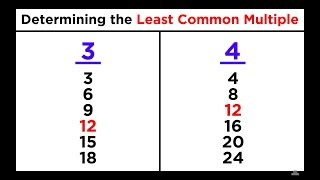17 Least Common Multiple (LCM)
Least Common Multiple (LCM)
483318 Adding & Subtracting Integers
Math Antics - Adding & Subtracting Integers
5154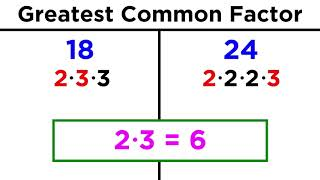18 Greatest Common Factor (GCF)
Greatest Common Factor (GCF)
3761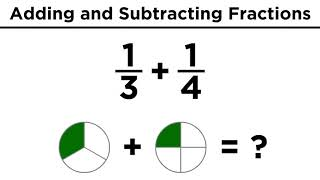19 Addition and Subtraction of Fractions
3762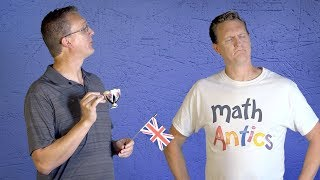20 Intro To Exponents (aka Indices)
Multiplication shows repeated addition and exponents show repeated multiplication.
5066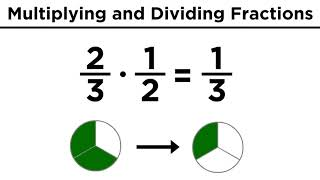20 Multiplication and Division of Fractions
Multiplication and Division of Fractions
3764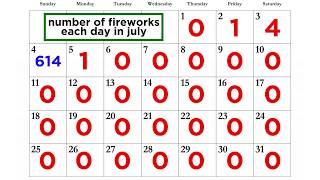21 Analyzing Sets of Data: Range, Mean, Median, and Mode
The median and the mode dilute the impact of anomalous data.
4938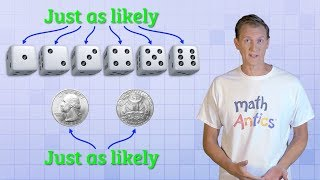23 Basic Probability
Math Antics - Basic Probability
5069-